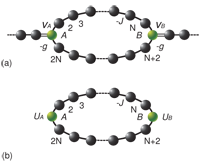January 2011
Mon Tue Wed Thu Fri Sat Sun
« Dec   Feb »
12
3456789
10111213141516
17181920212223
24252627282930
31

## The Black-Scholes Equation and Certain Quantum Hamiltonians

Juan M. Romero, O. Gonzalez-Gaxiola, J. Ruiz de Chavez, R. Bernal-Jaquez

Abstract: In this paper a quantum mechanics is built by means of a non-Hermitian momentum operator. We have shown that it is possible to construct two Hermitian and two non-Hermitian type of Hamiltonians using this momentum operator. We can construct a generalized supersymmetric quantum mechanics that has a dual based on these Hamiltonians. In addition, it is shown that the non-Hermitian Hamiltonians of this theory can be related to Hamiltonians that naturally arise in the so-called quantum finance.

http://arxiv.org/abs/1002.1667
Mathematical Physics (math-ph); High Energy Physics – Theory (hep-th); Quantum Physics (quant-ph)

## An exactly solvable quantum-lattice model with a tunable degree of nonlocality

Miloslav Znojil

An array of N subsequent Laguerre polynomials is interpreted as an eigenvector of a non-Hermitian tridiagonal Hamiltonian $H$ with real spectrum or, better said, of an exactly solvable N-site-lattice cryptohermitian Hamiltonian whose spectrum is known as equal to the set of zeros of the N-th Laguerre polynomial. The two key problems (viz., the one of the ambiguity and the one of the closed-form construction of all of the eligible inner products which make $H$ Hermitian in the respective {\em ad hoc} Hilbert spaces) are discussed. Then, for illustration, the first four simplest, $k-$parametric definitions of inner products with $k=0,k=1,k=2$ and $k=3$ are explicitly displayed. In mathematical terms these alternative inner products may be perceived as alternative Hermitian conjugations of the initial N-plet of Laguerre polynomials. In physical terms the parameter $k$ may be interpreted as a measure of the “smearing of the lattice coordinates” in the model.

http://arxiv.org/abs/1101.1183
Mathematical Physics (math-ph); Quantum Physics (quant-ph)

## Study of classical mechanical systems with complex potentials

A. Sinha, D. Dutta, P. Roy

We apply the factorization technique developed by Kuru and Negro [Ann. Phys. 323 (2008) 413] to study complex classical systems. As an illustration we apply the technique to study the classical analogue of the exactly solvable PT symmetric Scarf II model, which exhibits the interesting phenomenon of spontaneous breakdown of PT symmetry at some critical point. As the parameters are tuned such that energy switches from real to complex conjugate pairs, the corresponding classical trajectories display a distinct characteristic feature – the closed orbits become open ones.

http://arxiv.org/abs/1101.0909
Quantum Physics (quant-ph)
Physics Letters A : vol. 375 (2011) p 452-457

## Cryptohermitian Hamiltonians on graphs. II. Hermitizations.

Miloslav Znojil

Non-hermitian quantum graphs possessing real (i.e., in principle, observable) spectra are studied via their discretization. The discretized Hamiltonians are assigned, constructively, an elementary pseudometric and/or a more complicated metric. Both these constructions make the Hamiltonian Hermitian, respectively, in an auxiliary (Krein or Pontryagin) vector space or in a less friendly (but more useful) Hilbert space of quantum mechanics.

http://arxiv.org/abs/1101.1015
Quantum Physics (quant-ph); Mathematical Physics (math-ph)

## A physical interpretation for the non-Hermitian Hamiltonian

L. Jin, Z. SongWe explore a way of finding the link between a non-Hermitian Hamiltonian and a Hermitian one. Based on the analysis of Bethe Ansatz solutions for a class of non-Hermitian Hamiltonians and the scattering problems for the corresponding Hermitian Hamiltonians. It is shown that a scattering state of an arbitrary Hermitian lattice embedded in a chain as the scattering center shares the same wave function with the corresponding non-Hermitian tight binding lattice, which consists of the Hermitian lattice with two additional on-site complex potentials, no matter the non-Hermitian is broken PT symmetry or even non-PT. An exactly solvable model is presented to demonstrate the main points of this article.

http://arxiv.org/abs/1101.0351
Quantum Physics (quant-ph)